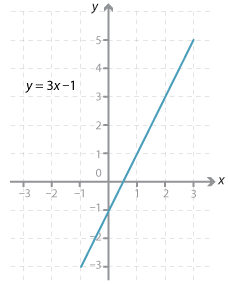## The y-intercept and gradient of a line

### The y-intercept

The y-intercept of a line is the y-value of the point where the line cuts the y-axis. The x-coordinate of every point on the y-axis is 0. So to find the y-intercept, we substitute x = 0 into the equation.

#### Example 1

Find the y-intercept of the line with equation y = 3x – 1.\begin{align}\text{When}\  x &= 0,\\ y &= 3 × 0 – 1\\      &=\ –1\end{align}

The y-intercept is –1.